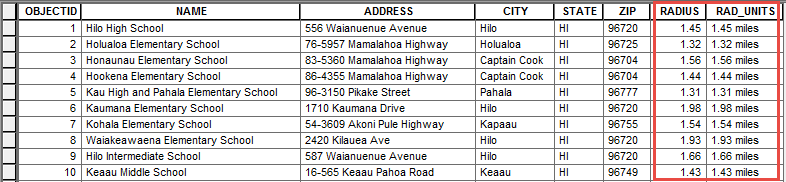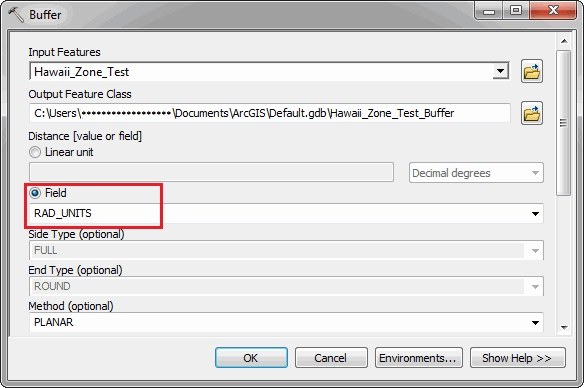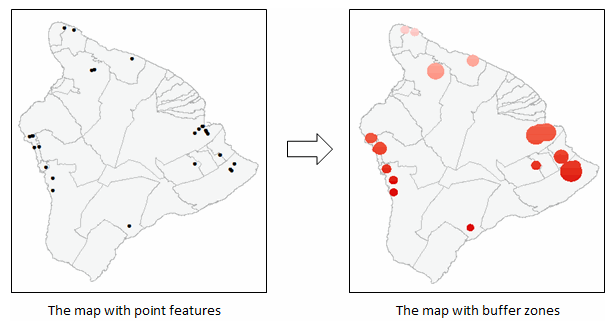English

# How To: Create buffers with different radii for point features using linear units

## Summary

When drawing point features on a map, buffers can be created around individual points to represent zonal areas within the defined radii of the points. The buffer radius for each point may vary in distance depending on the data.

The instructions provided describe how to apply buffers around point features to display zonal areas of varying radius distances in a linear unit using the Buffer (Analysis) tool.

## Procedure

1. In ArcMap, set the spatial reference of the layer using a projected coordinate system.  The projected coordinate system uses linear units of measurements such as feet, inches, meters, kilometers, and miles. The geographic coordinate system uses angular units, which are decimal degrees.
```Note:
Selecting the most appropriate coordinate system is an essential step to obtain accurate results when using the Buffer tool. Use the Project tool to project the layer to a different spatial reference if necessary. Alternatively, use the Output Coordinate System environment setting to set a different coordinate system before running the Buffer tool so that the buffers are created using the specified coordinate system. For more information, refer to ArcGIS for Desktop Help: Output Coordinate System (Environment setting).  ```
1. Right-click the layer in the Table Of Contents, and select Open Attribute Table.
2. In the attribute table, select Add Field from the Table Options drop-down list.
3. Create a new text field to store the buffer radius of each point feature and the desired linear unit. In this example, the text field is named RAD_UNITS and the linear unit used is miles.
```Note:
If the linear unit is not defined, the distance is calculated using the base reference unit of the layer's spatial reference by default.```
1. Start an edit session.
2. Right-click the RAD_UNITS field, and select Field Calculator.
3. Select the Python parser, and type the following expression:
`str(!RADIUS!) + " miles"`
The expression above takes the numeric values from the RADIUS field, and inserts the values in the RAD_UNITS field as a string with miles as the desired linear unit. For more information on units supported in ArcGIS for Desktop, refer to the following document, ArcGIS for Desktop Help: About distance units and editing.1. Open the Buffer tool. In the Buffer window, for the Input Features, enter the input feature layer. For the Distance [value or field], select Field. From the drop-down list, select RAD_UNITS, and click OK.